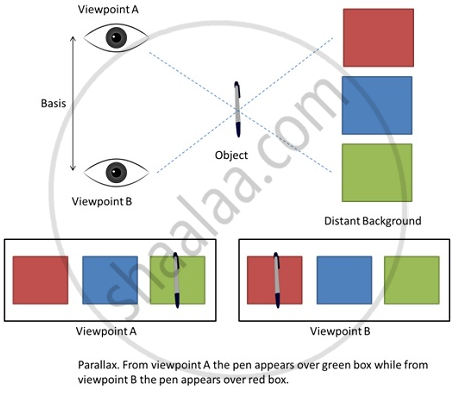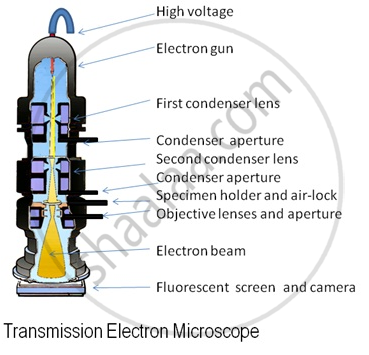# Measurement of Length

#### description

• Measurement of Large Distances - parallax angle or parallactic angle
• Estimation of Very Small Distances :  Size of a Molecule
• Range of Lengths

## Measurement of Length

Length can be measured using metre scale (10-3m to 102m), vernier callipers (10-4 m) and screw gauge and spherometer (10-5 m).

Measurement of Large Distances
Large distances such as the distance of a planet or a star from the earth cannot be measured directly with a metre scale. An important method in such cases is the parallax method.

Parallax is a displacement or difference in the apparent position of an object viewed along two different lines of sight, and is measured by the angle or semi-angle of inclination between those two lines. Distance between the two viewpoints is called Basis.

When you hold a pencil in front of you against some specific point on the background (a wall) and look at the pencil first through your left eye A (closing the right eye) and then look at the pencil through your right eye B (closing the left eye), you would notice that the position of the pencil seems to change with respect to the point on the wall. This is called parallax. The distance between the two points of observation is called the basis. In this example, the basis is the distance between the eyes.Measuring distance of a planet using parallax method
Measuring the distance of a far away planet:
Let us assume S is a planet a distance D from earth. A and B are two observatories on earth.
Distance                             AB = b
Parallax Angle  ∠ASB = θ
As the planet is very far away.
So,                                      b/D<< 1
and hence, θ is very small.

AB is an arc of circle with centre S and radius D
D = AS = BS
AB = b = Dθ, where θ is in radians
D = b/θ   ....(i)
After determining D, size or angular diameter at the planet can be determined using same method.
α = d/D   ....(ii)
Using these two equations, diameter of planet can be calculated.

Measuring very small distances
-To measure distances as low as size of a molecule, electron microscopes are used. These contain electrons beams controlled by electric and magnetic fields.

- Electron microscopes have a resolution of 0.6 Å or Angstroms.

- Electron microscopes are able to resolve atoms and molecules while using tunneling microscopy, it is possible to estimate size of molecule.Estimating size of molecule of Oleic acid :-
The steps followed in determining the size of molecule are:

• Dissolve 1 cm3 of oleic acid in alcohol to make a solution of 20 cm3.Take 1 cm3 of above solution and dissolve in alcohol to make a solution of 20 cm3 Concentration of oleic acid in the solution will be (1/(20x20)) cm3.

• Sprinkle lycopodium powder on the surface of water in a trough and put one drop of above solution. The oleic acid in the solution will spread over water in a circular molecular thick film.

• Measure the diameter of the above circular film using below calculations.

• If n – Number of drops of solution in water, V – Volume of each drop, t – Thickness of the film, A – Area of the film

Total volume of n drops of solution = nV cm3

Amount of Oleic acid in this solution = nV(1/(20 x 20)) cm3

Thickness of the film t = Volume of the film / Area of the film

t = nV/(20x20A) cm

Range of Lengths
The sizes of the objects we come across in the universe vary over a very wide range. These may vary from the size of the order of 10–14 m of the tiny nucleus of an atom to the size of the order of 1026 m of the extent of the observable universe. Table below gives the range and order of lengths and sizes of some of these objects

 Size of object or distance Length (m) Size of a proton 10-15 Size of atomic nucleus 10-14 size of hydrogen atom 10-10 Length of typical virus 10-8 Wavelength of light 10-7 Size of red blood corpuscle 10-5 Thickness of a paper 10-4 Height of the Mount Everest above sea level 104 Radius of the Earth 107 Distance of the moon from the Earth 108 Distance of the Sun from the Earth 1011 Distance of Pluto from the Sun 1013 Size of galaxy 1021 Distance to Andromeda galaxy 1022 Distance to the boundary of observable universe 1026

If you would like to contribute notes or other learning material, please submit them using the button below.